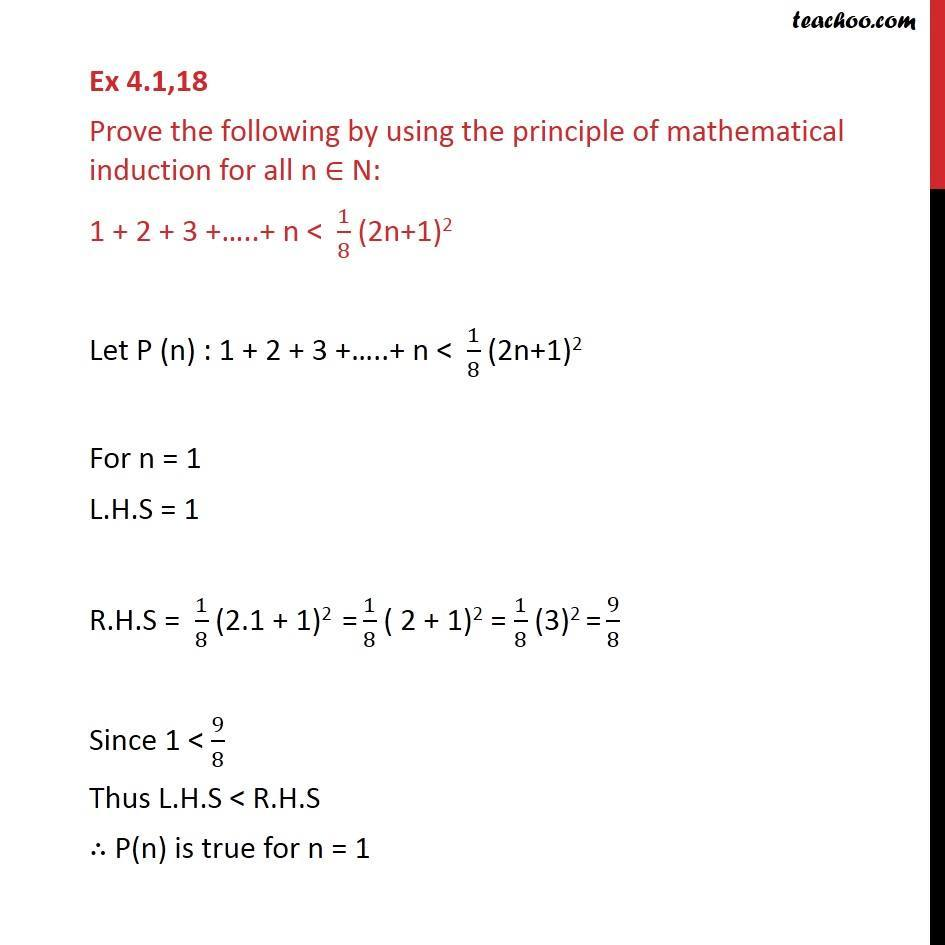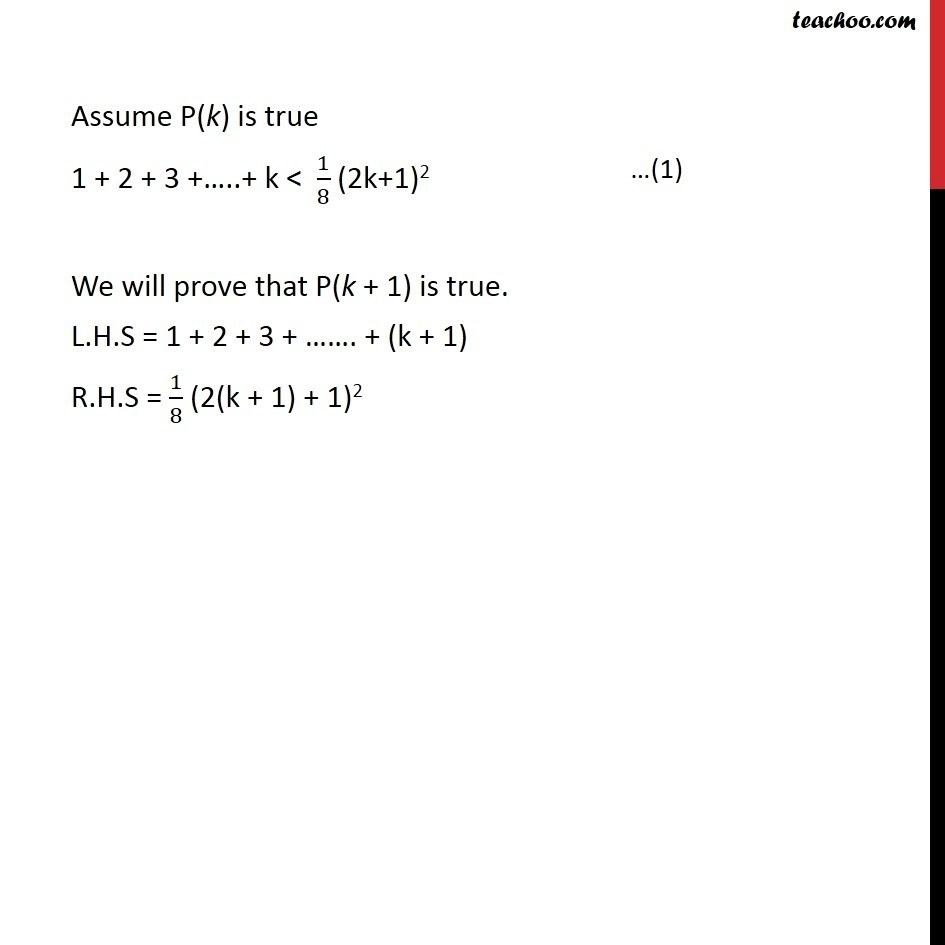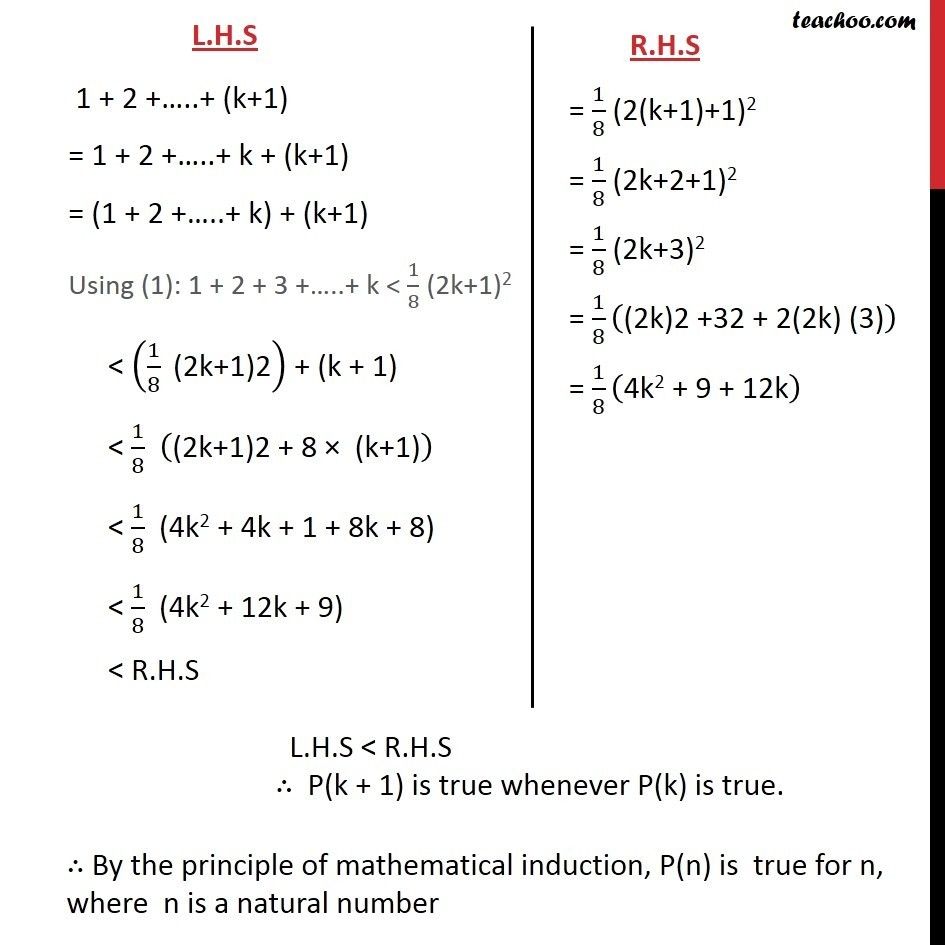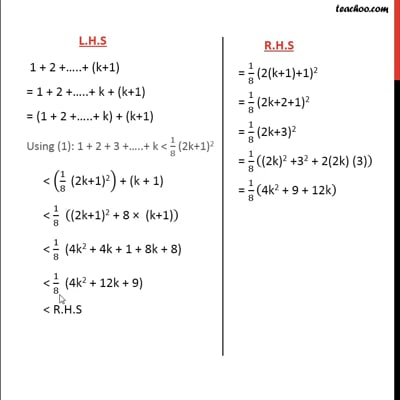Ex 4.1

Chapter 4 Class 11 Mathematical Induction
Serial order wiseThis video is only available for Teachoo black users

Solve all your doubts with Teachoo Black (new monthly pack available now!)

### Transcript

Ex 4.1,18 Prove the following by using the principle of mathematical induction for all n N: 1 + 2 + 3 + ..+ n < 1/8 (2n+1)2 Let P (n) : 1 + 2 + 3 + ..+ n < 1/8 (2n+1)2 For n = 1 L.H.S = 1 R.H.S = 1/8 (2.1 + 1)2 = 1/8 ( 2 + 1)2 = 1/8 (3)2 = 9/8 Since 1 < 9/8 Thus L.H.S < R.H.S P(n) is true for n = 1 Assume P(k) is true 1 + 2 + 3 + ..+ k < 1/8 (2k+1)2 We will prove that P(k + 1) is true. L.H.S = 1 + 2 + 3 + . + (k + 1) R.H.S = 1/8 (2(k + 1) + 1)2